Next: Slow and Fast Dynamos Up: Magnetohydrodynamic Fluids Previous: MHD Dynamo Theory

# Homopolar Generators

Some of the peculiarities of dynamo theory are well illustrated by the prototype example of self-excited dynamo action, which is the homopolar disk dynamo. As illustrated in Fig. 22, this device consists of a conducting disk which rotates at angular frequency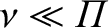about its axis under the action of an applied torque. A wire, twisted about the axis in the manner shown, makes sliding contact with the disc at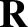, and with the axis at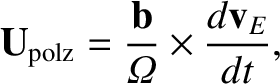, and carries a current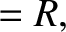. The magnetic field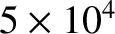associated with this current has a flux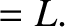across the disc, where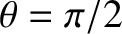is the mutual inductance between the wire and the rim of the disc. The rotation of the disc in the presence of this flux generates a radial electromotive force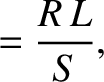(783)

since a radius of the disc cuts the magnetic flux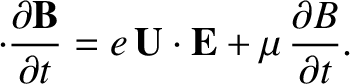once every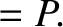seconds. According to this simplistic description, the equation for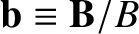is written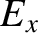(784)

where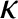is the total resistance of the circuit, and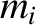is its self-inductance.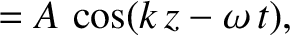Suppose that the angular velocityis maintained by suitable adjustment of the driving torque. It follows that Eq. (784) possesses an exponential solution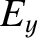, where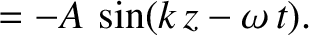(785)

Clearly, we have exponential growth of, and, hence, of the magnetic field to which it gives rise (i.e., we have dynamo action), provided that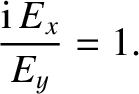(786)

i.e., provided that the disk rotates rapidly enough. Note that the homopolar generator depends for its success on its built-in axial asymmetry. If the disk rotates in the opposite direction to that shown in Fig. 22 then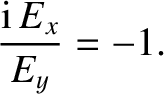, and the electromotive force generated by the rotation of the disk always acts to reduce. In this case, dynamo action is impossible (i.e.,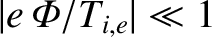is always negative). This is a troubling observation, since most astrophysical objects, such as stars and planets, possess very good axial symmetry. We conclude that if such bodies are to act as dynamos then the asymmetry of their internal motions must somehow compensate for their lack of built-in asymmetry. It is far from obvious how this is going to happen.

Incidentally, although the above analysis of a homopolar generator (which is the standard analysis found in most textbooks) is very appealing in its simplicity, it cannot be entirely correct. Consider the limiting situation of a perfectly conducting disk and wire, in which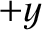. On the one hand, Eq. (785) yields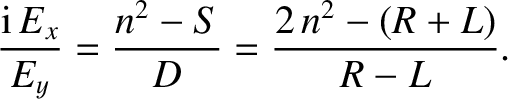, so that we still have dynamo action. But, on the other hand, the rim of the disk is a closed circuit embedded in a perfectly conducting medium, so the flux freezing constraint requires that the flux,, through this circuit must remain a constant. There is an obvious contradiction. The problem is that we have neglected the currents that flow azimuthally in the disc: i.e., the very currents which control the diffusion of magnetic flux across the rim of the disk. These currents become particularly important in the limit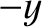.

The above paradox can be resolved by supposing that the azimuthal current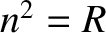is constrained to flow around the rim of the disk (e.g., by a suitable distribution of radial insulating strips). In this case, the fluxes through theand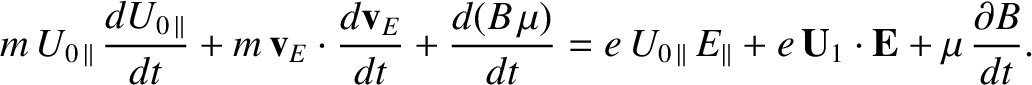circuits are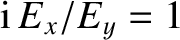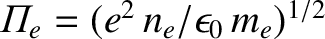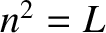(787)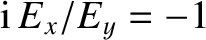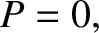(788)

and the equations governing the current flow are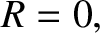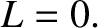(789)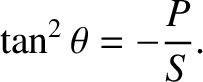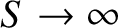(790)

where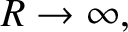, and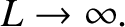refer to thecircuit. Let us search for exponential solutions,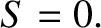, of the above system of equations. It is easily demonstrated that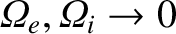(791)

Recall the standard result in electromagnetic theory that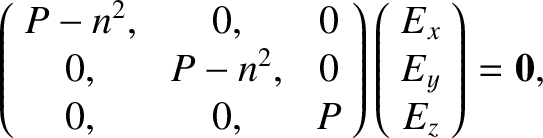for two non-coincident circuits. It is clear, from the above expression, that the condition for dynamo action (i.e.,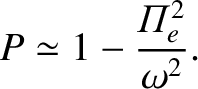) is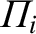(792)

as before. Note, however, that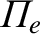as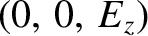. In other words, if the rotating disk is a perfect conductor then dynamo action is impossible. The above system of equations can transformed into the well-known Lorenz system, which exhibits chaotic behaviour in certain parameter regimes.It is noteworthy that this simplest prototype dynamo system already contains the seeds of chaos (provided that the formulation is self-consistent).

It is clear from the above discussion that, whilst dynamo action requires the resistance of the circuit,, to be low, we lose dynamo action altogether if we go to the perfectly conducting limit,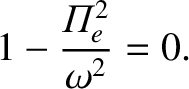, because magnetic fields are unable to diffuse into the region in which magnetic induction is operating. Thus, an efficient dynamo requires a conductivity that is large, but not too large.Next: Slow and Fast Dynamos Up: Magnetohydrodynamic Fluids Previous: MHD Dynamo Theory
Richard Fitzpatrick 2011-03-31